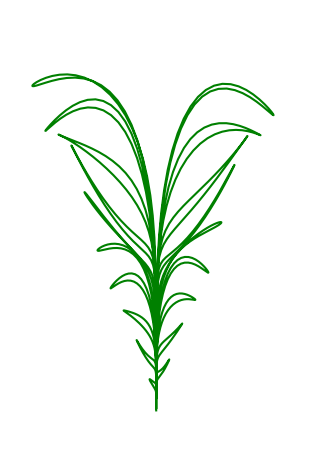# Simple equations whose plot looks like field grass

Math Overflow has an interesting question about unexpected mathematical images. Here’s a response from Payam Seraji that was easy to code up.Here’s the code that produced the image.

```from numpy import *
import matplotlib.pyplot as plt

t = linspace(0, 39*pi/2, 1000)
x = t*cos(t)**3
y = 9*t*sqrt(abs(cos(t))) + t*sin(0.2*t)*cos(4*t)
plt.plot(x, y, c="green")
plt.axes().set_aspect(0.3)
plt.axis('off')
```